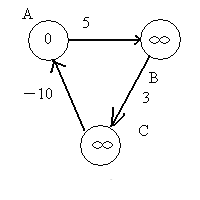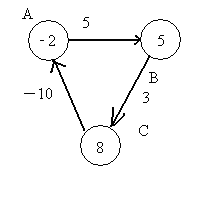# 浅谈路径规划算法之Bellman-Ford算法

最近在研究AGV系统的调度算法，为了实现多AGV小车的运行，给每一个AGV小车规划一条最优路径，对比了Bellman-Ford算法、SPFA算法、Dijkstra算法、Floyd算法和A*算法的优缺点，最终确定了使用A*算法作为路径规划算法。

1Bellman-Ford算法

1）贝尔曼-福特算法是计算从源点到任意一点的最短路径的长度，初始化数组dist0dist[i]为无穷大。

2）以下操作循环执行至多n-1次，n为顶点数：

对于每一条边 edge(Start,End)，如果dist[Start] +Weight(Start, End)<dist[End]，则令dist[End] =dist[Start]+Weight(Start, End)。若上述操作没有对dist进行更新，说明最短路径已经查找完毕，或者部分点不可达，跳出循环。否则执行下次循环；

注释：Start是一条有向边的起点，End是一条有向边的终点；edge(Start,End)为节点Start和节点End之间边；Weight(Start, End)为节点Start和节点End之间的边的权值；dist[i]是指源节点到i节点的距离；

BellmanFord算法可以大致分为三个部分

！！！之所以需要第三部分的原因，是因为，如果存在从源点可达的权为负的回路。则 应为无法收敛而导致不能求出最短路径。#include <iostream>
#include <stdio.h>
using namespace std;

const int maxnum=100;   //最大边数
const int maxint=9999;  //源点和某点不可达时的距离

//有向边的结构体
typedef struct Edge
{
int Start; //有向边边的起始点
int End;   //有向边的终点
int Weight;//边的权重
}Edge;

Edge edge[maxnum];//有向边的数组
int dist[maxnum]; //距离数组

//节点的数目、边的数目、源节点的下标
int nodenum,edgenum,source;

//初始化函数
void Init()
{
cin>>nodenum>>edgenum>>source;

for(int i=1;i<=nodenum;i++)
dist[i]=maxint;

dist[source]=0;

for(int i=1;i<=edgenum;i++)
{
cin >> edge[i].Start >> edge[i].End >> edge[i].Weight;
if(source==edge[i].Start)
{
dist[edge[i].End]=edge[i].Weight;
}
}
}

//松弛函数
void Relax(int Start,int End,int Weight)
{
if(dist[End]>dist[Start]+Weight)
dist[End]=dist[Start]+Weight;
}

//贝尔曼福特函数
bool Bellman_Ford()
{
for(int i=1;i<=nodenum-1;i++)
{
for(int j=1;j<=edgenum;j++)
{
Relax(edge[j].Start,edge[j].End,edge[j].Weight);
}
}
bool flag=1;
for(int i=1;i<=edgenum;i++)
{
//判断是否存在负回路
if(dist[edge[i].End]>dist[edge[i].Start]+edge[i].Weight)
{
flag=0;
break;
}
}
return flag;
}

int main()
{
freopen("out.txt","r",stdin);//打开txt
Init();
if(Bellman_Ford())
{
for(int i=1;i<nodenum;i++)
cout<<dist[i]<<endl;//打印源节点到每个节点的距离
}
return 0;
}

贝尔曼算法的时间复杂度是O(V*E)，是非常大的，所以它的效率非常低。

08-254110
10-14
07-271万+
07-072万+
10-241万+
02-286330
04-16723
09-193951
08-242988
08-106701
07-301792
04-2671
07-08941
07-062008
01-2937
11-13211
10-11916

### “相关推荐”对你有帮助么？

•非常没帮助
•没帮助
•一般
•有帮助
•非常有帮助被折叠的  条评论 为什么被折叠?到【灌水乐园】发言1234565623

¥2 ¥4 ¥6 ¥10 ¥20余额支付 (余额：-- )扫码支付获取中扫码支付点击重新获取扫码支付1.余额是钱包充值的虚拟货币，按照1:1的比例进行支付金额的抵扣。
2.余额无法直接购买下载，可以购买VIP、C币套餐、付费专栏及课程。余额充值